High Level Seating Arrangement Questions For SBI PO PDF

0
1075high level seating arrangement questions for sbi po pdf

High Level Seating Arrangement Questions For SBI PO PDF

High Level Seating Arrangement Questions for SBI PO Prelims and Mains exam. Download SBI PO High Level Seating Arrangement questions on with solutions.

Instructions

Read the following and answer the questions.

Eight friends – A, B, C, D, E, F, G and H, not necessarily in the same order sit around a square table. Four of the friends sit at the corners, while the other four sit at the middle of each side. The ones sitting in the middle face away from the center while those sitting in the corners face the center.

1. G sits at one of the corners.
2. Both the neighbours of D face away from the center.
3. Both the neighbours of B face the center.
4. F sits to the immediate right of D.
5. H sits third to the left of E.
6. D does not sit opposite G.
7. B sits second to the right of F.
8. A and F sit opposite each other.

Question 1: How many seating arrangements are possible?

a) 1

b) 2

c) 3

d) 4

e) 5

Instructions

Study the following information carefully and answer the given questions.

Seven friends P, Q, R, S, T, U and V are sitting in a straight line facing the north direction. Further it is known that:
1) V is second to the right of T
2) P is third to the left of R
3) T is to the right of U
4) Q is third to the left of U

Question 2: How many different seating arrangements are possible?

a) 1

b) 2

c) 3

d) 4

e) 5

Instructions

Read the below information carefully and answer the following questions.

12 seats are arranged in two rows such that there are six seats in each row. The seats are placed in such a manner that the distance between two adjacent chairs is same and every chair has exactly one chair in the opposite row facing it. A, B, C, D, E, and F sit in the first row while P, Q, R, S, T, and U sit in the second row. The following information is also known:
1) D is sitting opposite Q
2) F is sitting opposite T
3) U is not sitting adjacent to Q
4) B is sitting third to the right of D
5) T is not sitting adjacent to Q
6) Neither Q nor R is sitting at an extreme end
7) E is to the right of A
8) B is sitting opposite R
9) C and F are sitting adjacent to each other

Question 3: How many unique arrangements are possible?

a) 1

b) 2

c) 3

d) 4

e) 5

Instructions

Read the following information carefully to answer the questions that follow.
Eight dancers- M, N, O, P, Q, R, S, and T –are sitting around a square table such that four people are sitting on the corners of the table while the other four sit on the middle of each edge of the table. The people who are sitting on the corners are facing the centre of the table while people who are sitting on the edges face outward.
Further, the following information is also known about their seating arrangement.

(i)N is at one of the corners.
(ii) S and M face opposite directions but do not face each other.
(ii) R is the left neighbour of N.
(iii) T is two places to the right of M.
(iv) O and P do not face each other.
(v) P and Q do not face each other.
(vi) Q is closer to S to its right that of its left.

Question 4: Which of the following pairs are neighbors in the given arrangement as well as in the arrangement when arranged alphabetically?

a) M & T

b) R & S

c) P & Q

d) N & O

e) None of the above.

Instructions

Read the following data carefully to answer the questions that follow.
Eight friends- S, T, U, V, W, X, Y, and Z –are sitting around a square table such that four people are sitting on the corners of the table while the other four sit on the middle of each edge of the table. The people who are sitting on the corners are facing outwards while people who are sitting on the edges face the centre of the table.
Further the following information is also known about their seating arrangement.
(i)Y is at one of the corners.
(ii) U is 3 places to the right of X.
(iii) X is not facing anyone.
(iv) The person facing V is 2 places to the right of U
(v) S and Z face opposite directions but do not face eachother.
(vi) W is third to the left of Z.

Question 5: Which of the following pairs are neighbors in the given arrangement as well as in the arrangement when arranged alphabetically?

a) U and V

b) T and U

c) Z ans S

d) W and X

e) T and S

Instructions

Read the following information carefully and answer the questions which follow.
8 friends B,D,F,H,J,L,N,P are sitting in the first row of a movie theater facing the screen.
1)Neither F nor N is sitting at the extreme ends and they have 4 members between them.
2) F has only one member to his left.
3)The number of people to the left of P is same as the number of people to the right of J.
4) There are exactly 3 members to the left of D and B is only one sitting between D and L.

Question 6: How many seating arrangements are possible?

a) 2

b) 4

c) 8

d) 16

e) 32

Instructions

Eight friends A, B, C, D, E, F, G and H are sitting in a straight line facing the same direction.
Further, the following information is also known.
* C sits sixth to the right of B.
* F is a neighbor of C and sits second to the right of A.
* E sits next to the left of G.
* D is not a neighbor of A.
* A is not a neighbor of C.

Question 7: How many different seating arrangements are possible?

a) 1

b) 2

c) 3

d) 4

e) None of these

Instructions

Read the following information carefully and answer the questions that follow.

8 chairs are arranged in 2 rows, row 1 (seats 1-4 facing south) and row 2 (seats 5-8 facing north), such that seat 1 is facing seat 5 and vice-versa, seat 2 is facing seat 6 and vice-versa and so on. Pair of seats such as 1-8, 6-3 etc. are diagonally opposite to each other. Eight people A, B, C, D, E, F, G, and H are sitting on these chairs. The following information is known about their seating arrangement-
(i) A sits diagonally opposite to B and C sits opposite to D.
(ii) G doesn’t sit on the extreme.
(iii) If B sits opposite to G, H sits opposite to E. In all other cases, B sits opposite to E and G sits opposite to H.
(iv) G sits towards the left of A.
(v) B and H sit in the same row. Similarly, A and C sit in the same row.
(vi) A doesn’t sit adjacent to E and G doesn’t sit diagonally opposite to D.
(vii) B sits in row 1.

Question 8: How many unique arrangements are possible?

a) 8

b) 6

c) 4

d) 2

e) None of these

Instructions

A, B, C, D, E, F, G, H are standing in a row facing north.
1) F is between G and E with G to his immediate left and E to his immediate right.
2) G is not at any extreme end.
3) A is 6th to the left of E.
4) H is 6th to the right of C.

Question 9: If we arrange the persons in an alphabetical order in a row, then how many persons would have same position from the left?

a) 1

b) 2

c) 3

d) 4

e) Cannot be determined

Question 10: Who is sitting at the last position from the left?

a) D

b) A

c) H

d) E

e) Cannot be determined.

Question 11: If D is not the neigbor of G, then who is sitting immediately left of D?

a) B

b) A

c) G

d) C

e) None of these.

Question 12: Who is sitting at the second position from the left?

a) C

b) A

c) B

d) G

e) Cannot be determined

Question 13: How many seating arrangements are possible?

a) 1

b) 2

c) 3

d) 4

e) 5

Instructions

Study the following information to answer the given question:
Twelve people are sitting in two parallel rows containing six people each such that they are equidistant from each other. In row 1: P, Q, R, S, T and V are seated and all of them are facing South. In row 2: A, B, C, D, E and F are seated and all of them are facing North. Therefore in the given seating arrangement, each member is seated in a row faces another member of the other row.

• S sits third to the right of Q.
• Either S or Q sits at an extreme end of the line.
• The one who faces Q sits second to the right of E.
• Two people sit between B and F.
• Neither B nor F sits at an extreme end of the line.
• The immediate neighbour of B faces the person who sits third to the left of P.
• R and T are immediate neighbours
• C sits second to the left of A
• T does not face the immediate neighbour of D.

Question 14: Four of the following five are alike in a certain way based on the give arrangements and so form a group.Which is the one that does not belong to that group ?

a) A-T

b) B-T

c) F-P

d) C-V

e) E-Q

From 1, we know that G sits at one of the corners, so let’s fix G at 1.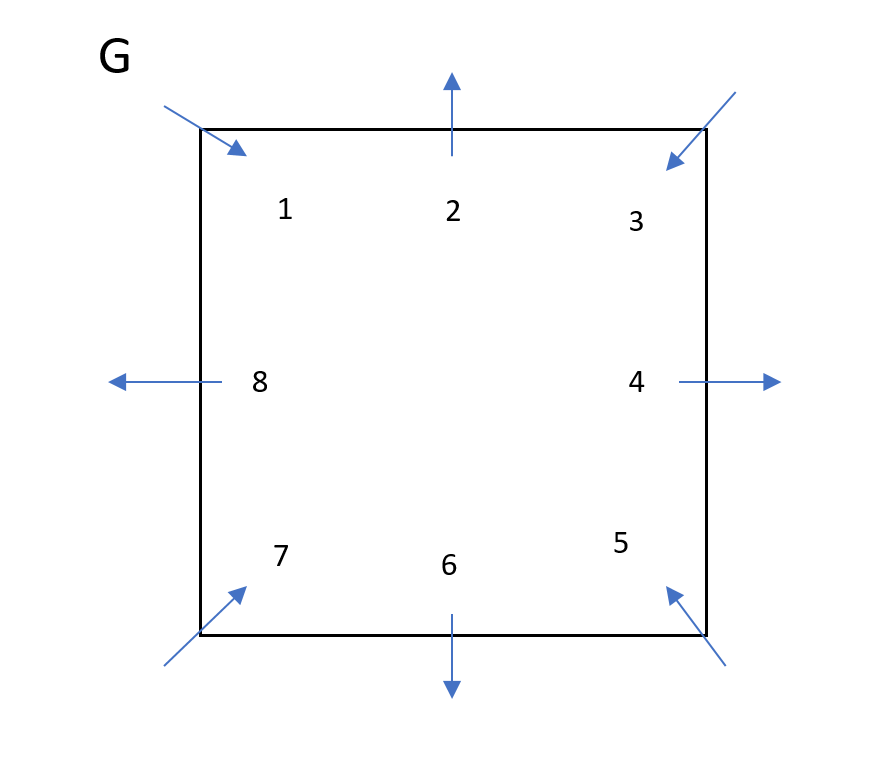From 2 and 6, we know that D sits at one of the corners but does not sit opposite G.
D can sit at either 3 or 7.

If D sits at 7:
From 4, F sits at 6. From 8, A sits opposite F, so A sits at 2.
From 7, B sits second to the right of F, thus B sits at 8.
From 5, H sits third to the left of E, but the only vacant places are 3, 4 and 5. There is no way to place H and E, thus, this case is not possible.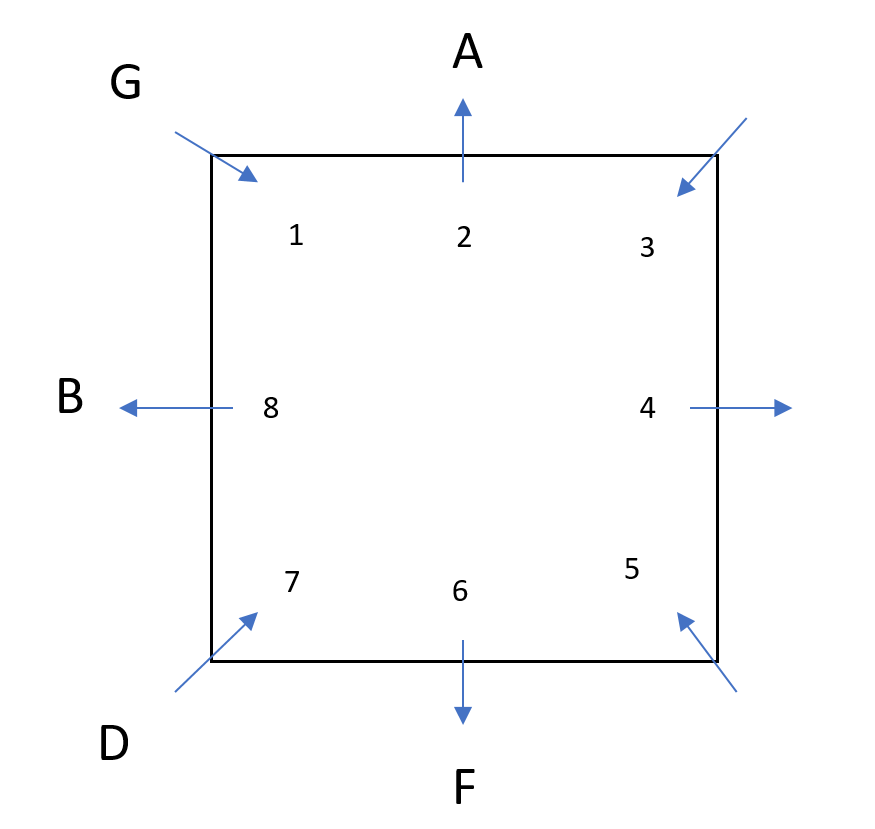If D sits at 3:
From 4, F sits to the immediate right of D, thus, F sits at 2.
From 8, A sits opposite A, so A sits at 6.
From 5, H sits third to the left of E, there are two ways possible – E sits at 5 and H at 8 or E at 8 and H at 5.
From 7, B sits at 4.
C will occupy the only vacant place 7.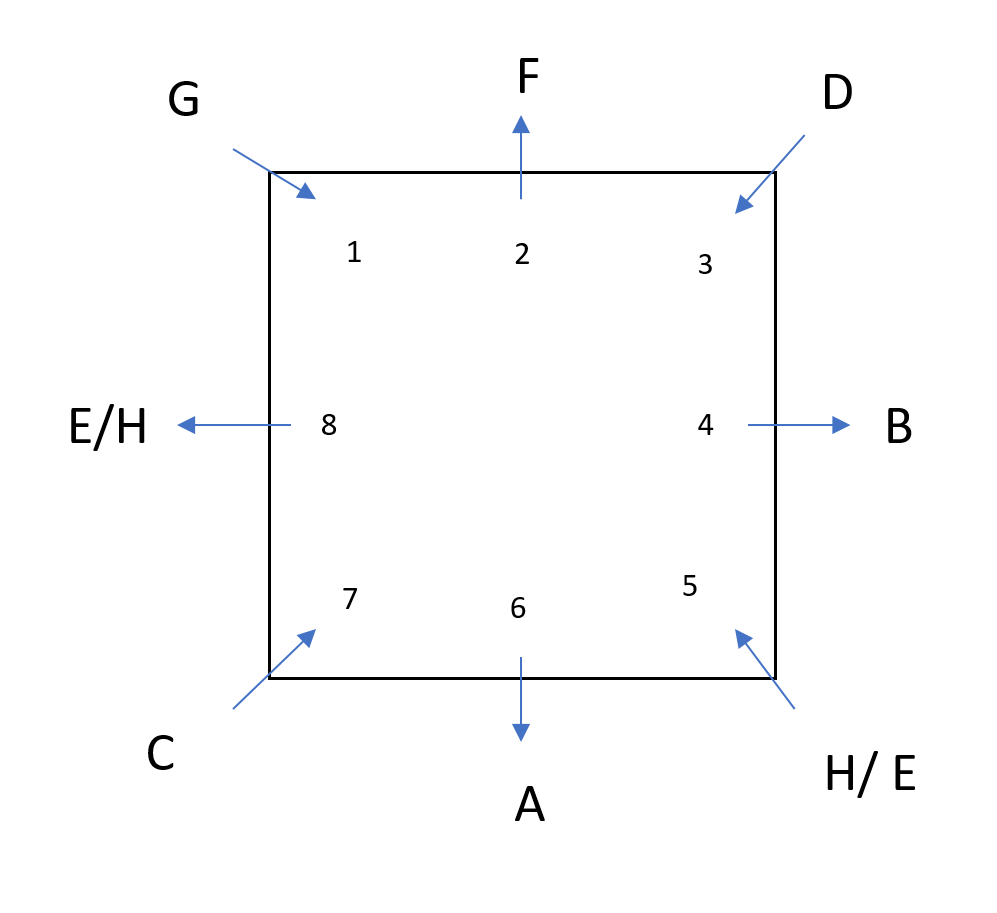Since H and E can interchange their positions, two arrangements are possible. Option B is the correct answer.

Let us number the seating positions 1 to 7 from left to right as shown.
From statements 4 and 1 we know that Q is third to the left of U and V is second to the right of T.
But from statement 3 we know that T is to the right of U.
This is possible only when (Q, U, T, V) are sitting in positions (1, 4, 5, 7) in that order.
Now from statement 2 we know that P is third to the left of R. This means that P and R must be sitting in positions 3 and 6 respectively.
Now the only remaining person S must be sitting in position 2.
Hence the seating arrangement will be as shown below.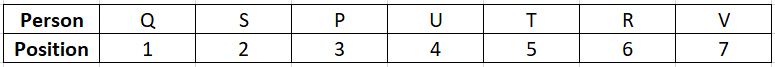Let us number the positions in row 1 from 1 to 6 and in row 2 from 7 to 12 as shown in the figure below.From the fourth statement we know that B sits third to the right of D.
From first and eighth statements we know that B is opposite R and D is opposite Q.
From statement 6 we know that neither R nor Q sits at an extreme end. ‘
This means that neither B nor D sits at an extreme end.
From this we understand that B and D sit in positions 2 and 5 respectively.
This means that R and Q sit in positions 8 and 11 respectively.
From statement 9 we know that C and F sit adjacent to each other. Thus they must be sitting in positions 3 and 4 in any order.
From statements 2 and 5 we know that F is sitting opposite T who is not adjacent to Q.
This means that F and C must be sitting in positions 3 and 4 respectively and T must be sitting in position 9.
From statement 7 we know that E and A must be sitting in positions 1 and 6 respectively.
Now from statement 3 we know that U must be in position 7.
Now the only remaining persons P and S can be sitting in positions 10 and 12 in any order.
Thus the final seating arrangement is as shown below.Let us number the positions as shown in the diagram.
N is at one of the corners(given). Let us place N in position 1.
R is the left neighbour of N(given). R should come in position 8.
S and M face opposite directions but do not face each other (given). Hence, S and M must be at the edges of the table (positions 2 and 6 are the only possible positions left for them).
T is two places to the right of M(given). Hence, T should be in position 4.
3,5,7 are the only positions left and O, P, Q are the only people left to occupy them.
Given that, O and P do not face each other, P and Q do not face each other.
Hence, O and Q should face each other and P should be in position 5.
Q is closer to S to its right that of its left (given). Hence, Q will be in position 7.
The following is the final arrangement.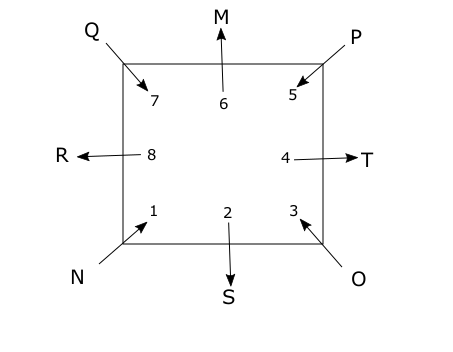The arrangement when arranged alphabetically looks as shown below.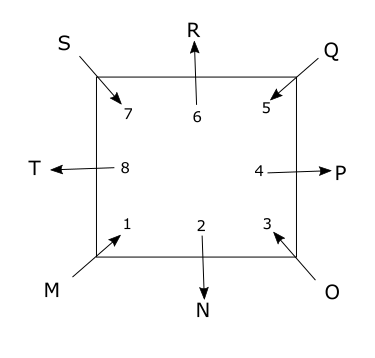None of the given pairs are neighbors in both the arrangements.
Hence, option E is the correct answer.

Let us number the positions as shown in the diagram.
Y is at one of the corners.(given) let us place Y in position 1.
S and Z face opposite directions but do not face each other(given). Hence, S and Z must be at the opposite corners(positions 1 and 7).
X is not facing anyone(given), X must be at a corner and should be facing Y.
Hence X will be in position 5.
U is 3 places to the right of X(given). Hence, U will be in position 2.
The person facing V is 2 places to the right of U(given). Hence, V should be in position 8.
W is third to the left of Z.(given). If Z is in position 7 there will not be any position left for W to occupy. Hence, Z should be in position 3 and S in position 7.
The only position left is 4 and it will be occupied by T.
The following is the final arrangement.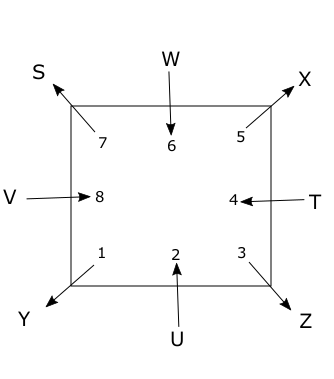The following is the order when arranged alphabetically.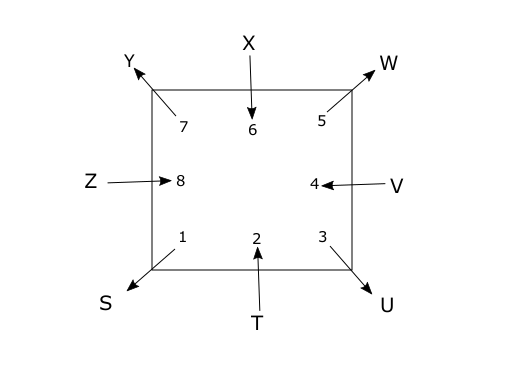In both the arrangements W and X are neighbors.
Hence, option D is the correct answer.

Let us number the positions as shown in the diagram.
Neither F nor N is sitting at the extreme ends and they have 4 members between them.(given).Therefore F andN will be in positions 2 and 7.
F has only one member to his left(given), F will be in position 2 and N will be in position 7.
There are exactly 3 members to the left of D(given), D will be in position 4.
B is only one sitting between D and L(given), B and L can only be in position 5 and 6 respectively.
The Number of people to the left of P is same as the number of people to the right of J(given), P and J will be in position 1 and 8 as there are no other positions available for them.
H will occupy the position 3.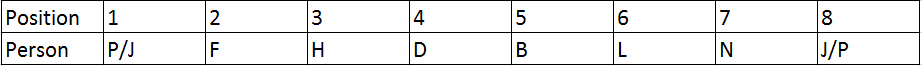There are two different seating arrangements possible.

Let’s number the positions as shown below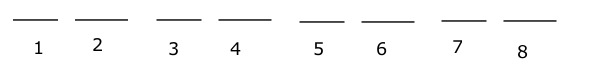“C sits sixth to the right of B.” ==> two arrangements possible C and B on 8th, 2nd spot respectively or 7th, 1st spot respectively.

Case 1: B and C on spots 2 and 8

“F is a neighbor of C and sits second to the right of A.” ==> F and A sit on spots 7 and 5 respectively

“E sits next to the left of G.” ==> the only possible arrangement is, E and G on spots 3 and 4 respectively.

“D is not a neighbor of A.” ==> D on spot 1.

the following arrangement could be derived in this case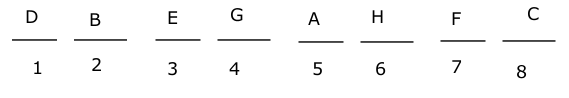Case 2: B and C on spots 1 and 7 respectively.

“F is a neighbor of C and sits second to the right of A.”, “A is not a neighbor of C” ==> F and A sit on spots 6 and 4 respectively

“E sits next to the left of G.” ==> the only possible arrangement is, E and G on spots 2 and 3 respectively.

“D is not a neighbor of A.” ==> D on spot 8.

the following arrangement could be derived in this case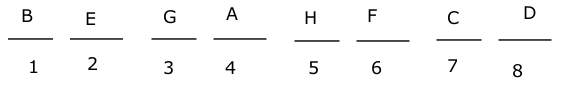Two seating arrangements are possible.

From (vii) we know that B sit in row 1 and from (i) we know that he sits diagonally opposite to A. So, B can sit on seat 1 or seat 2. He can’t sit on 3 as in that case A will sit on 6 and G on 5 which can’t be possible as he doesn’t sit on extreme. For the same reason, B on seat 4 is also not possible.
Case 1: B sits on seat 1
This means that A sits on 8. In this case B can’t sit opposite to G. So, from (iii) he sits opposite to E and H sits opposite to G. Also, H must be in row 1. So, G sits opposite to H and C opposite to D. So, G and C must be seated on either 6 or 7. But in both the cases, G will be diagonally opposite to D.Case 2: B sits on seat 2
This means A is seated on 7. So, only place left for G is 6 as he can’t sit on extreme. So, in this case B sits opposite to G, this means H sits opposite to E. C and D also sit opposite to each other. This, means A must be seated opposite to F. Since, A doesn’t sit adjacent to E, C must be seated on 8. This means that D is on 4. Since H sits in the same row as B, he sits on 1 and E on 5. The complete arrangement is as shown below-We can see that only one arrangement is possible.

From the first point, we know that GFE are sitting on consecutive seats. A is 6th to the left of E means E should occupy either 7th or 8th position.
The possibilities are
– A – – – G F E or
A – – – G F E –
Now H is 6th to the right of C.
This case can be satisfied by only second arrangement.
A C – – G F E H.
There are two possible seating arrangements:A C B D G F E H or A C D B G F E H.
If we arrange A B C D E F G H, then A, D, H are at the same place in the first arrangement and A, H are in the same place in the second arrangement.
The number can be 2 or 3. Hence the answer cannot be determined.

From the first point, we know that GFE are sitting on consecutive seats. A is 6th to the left of E means E should occupy either 7th or 8th position.
The possibilities are
– A – – – G F E or
A – – – G F E –
Now H is 6th to the right of C.
This case can be satisfied by only second arrangement.
A C – – G F E H.
There are two possible seating arrangements:A C B D G F E H or A C D B G F E H.

From the first point, we know that GFE are sitting on consecutive seats. A is 6th to the left of E means E should occupy either 7th or 8th position.
The possibilities are
– A – – – G F E or
A – – – G F E –
Now H is 6th to the right of C.
This case can be satisfied by only second arrangement.
A C – – G F E H.
There are two possible seating arrangements:A C B D G F E H or A C D B G F E H.
The given case is of ACDBGFEH. C is to the immediate left of D.

From the first point, we know that GFE are sitting on consecutive seats. A is 6th to the left of E means E should occupy either 7th or 8th position.
The possibilities are
– A – – – G F E or
A – – – G F E –
Now H is 6th to the right of C.
This case can be satisfied by only second arrangement.
A C – – G F E H.
There are two possible seating arrangements:A C B D G F E H or A C D B G F E H.

From the first point, we know that GFE are sitting on consecutive seats. A is 6th to the left of E means E should occupy either 7th or 8th position.
The possibilities are
– A – – – G F E or
A – – – G F E –
Now H is 6th to the right of C.
This case can be satisfied by only second arrangement.
A C – – G F E H.
There are two possible seating arrangements:A C B D G F E H or A C D B G F E H.

From the first three conditions, two solns are possible

S _ _ Q _ _

_ E _ _ _ _

or

_ _ S _ _ Q

_ _ _ E _ _

As B and F have two people between them and neither are at the extremes, they must by in the second and fifth place. Hence, we can rule out configuration 1.

From the 6th condition, B should be fifth from left and F second.

Thus, we get

P _ S _ _ Q

_ F _ E B _

As C is second to the left of A, C must be first from left and A third. Hence, D is last from left. Thus, we can place all the people as follows

$\downarrow$ P V S T R Q

$\uparrow$ C F A E B D

In all pairs except E-Q, the first person faces an immediate neighbour of the second person.

Hence, option E is correct.